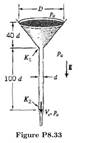# Under the action of gravity, water of density ρ passes steadily through a circular funnel into a vertical pipe of diameter d, and then exits from the pipe, falling freely under gravity, as shown in Figure P8.33. Atmospheric pressure acts everywhere outside the funnel and pipe. The entrance to the pipe has a loss coefficient K1 = 0.5, the pipe has a friction factor f = 0.01, and the exit from the pipe has a loss coefficient K2 = 1.0. The kinetic energy coefficient α = 1. Find the outlet velocity in terms of g and d. You may assume that D ≫ d.

Question
1 views

Under the action of gravity, water of density ρ passes steadily through a circular funnel into a vertical pipe of diameter d, and then exits from the pipe, falling freely under gravity, as shown in Figure P8.33. Atmospheric pressure acts everywhere outside the funnel and pipe. The entrance to the pipe has a loss coefficient K1 = 0.5, the pipe has a friction factor f = 0.01, and the exit from the pipe has a loss coefficient K2 = 1.0. The kinetic energy coefficient α = 1. Find the outlet velocity in terms of g and d. You may assume that D ≫ d.### This question hasn't been answered yet.